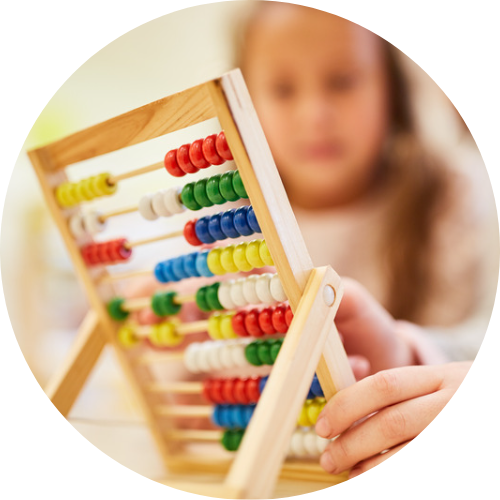# Mathematics

Primary School Mathematics is an educational program that aims to develop students’ mathematical thinking skills and teach them basic mathematical concepts. This course covers topics such as numbers, operations, measurement units, geometry and data analysis. It also gives students opportunities to enhance their mathematical problem-solving skills.

This course will run for 32 weeks, using online activities, videos, assignments and in-class activities that encourage students to learn interactively with age-appropriate mathematics materials. Through these activities, students will gain a better understanding of mathematical concepts, develop various strategies for solving mathematical problems and improve their mathematical skills through practice.

In the lessons, students will learn basic arithmetic operations (addition, subtraction, multiplication, division) using learning materials. They will also receive information about different measurement units and geometric shapes. Data collection and analysis skills are also included in these lessons.

Primary School Mathematics equips students with mathematical skills that they can use throughout their lives. The development of mathematical thinking skills helps students comprehend problems, generate solutions and think from a wider perspective. This course, which will last for 32 weeks, offers a valuable opportunity for students to improve their mathematical skills and use mathematics more effectively in their daily lives.